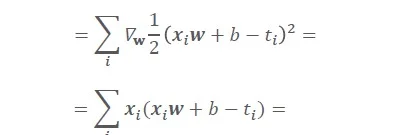11 Aug 2023

Posted on:

07 Jan 2023

0

# Can some one help to explain this step?Posted on:

11 Aug 2023

0

Ignoring the summation because that's in both lines, work the problem from there.

In the top line you're taking the derivative with respective w of` 1/2*(x*w+b-t)^2`
If you focus on the bit in parenthesis we can expand it manually. It's a bit of work, but we'll get:
`w^2*x^2 + 2*w*x*b - 2*w*x*t - 2*b*t + t^2 + b^2`

So we have, multiplied by 1/2 and with a derivative with respect to w. We'll move the 1/2 out of the way for now because it doesn't affect the derivative, and we'll just take the derivative of that line above. It's a polynomial so we drop any terms that don't have a w, and for any terms that do have a w we lower the order of the exponent on w and multiply term by that exponent. All of the exponents on w are either 1 or 2 so we're left with:
`2*w*x^2 + 2*x*b - 2*x*t`

Now we have that 1/2 from earlier, which quite nicely cancels out all of the factors of 2:
`w*x^2+ x*b - x*t`

And lastly, we see that all of the terms have a multiplcation of x, so we can factor that out:
x * (w*x + b - t)

Hopefully going step by step clears that up. The two keys are 1) expanding the parenthesis squared, and 2) knowing the rules derivation (moving the 1/2 and take derivates of w and w^2)